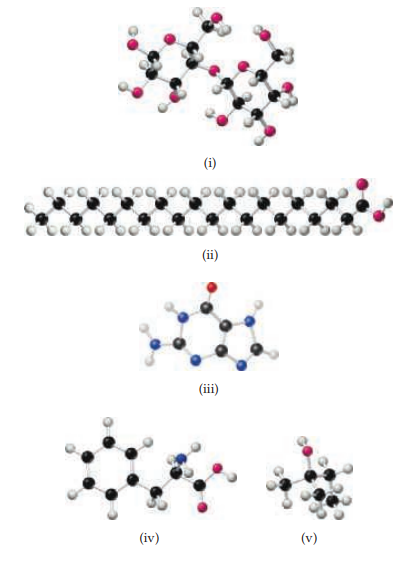×
Get Full Access to Chemistry: The Central Science - 14 Edition - Chapter 24 - Problem 24.6
Get Full Access to Chemistry: The Central Science - 14 Edition - Chapter 24 - Problem 24.6

×

# ?From examination of the molecular models i–v, choose the substance that (a) can be hydrolyzed to form a solution containing glucose,ISBN: 9780134414232 1274

## Solution for problem 24.6 Chapter 24

Chemistry: The Central Science | 14th Edition

• Textbook Solutions
• 2901 Step-by-step solutions solved by professors and subject experts
• Get 24/7 help from StudySoup virtual teaching assistantsChemistry: The Central Science | 14th Edition

4 5 1 270 Reviews
16
0
Problem 24.6

From examination of the molecular models i–v, choose the substance that

(a) can be hydrolyzed to form a solution containing glucose,

(b) is capable of forming a zwitterion,

(c) is one of the four bases present in DNA,

(d) reacts with an acid to form an ester,

(e) is a lipid. [Sections 24.6–24.10]Step-by-Step Solution:

Step 1 of 5) We first combine the carboxyl group of alanine with the amino group of glycine to form a peptide bond and then the carboxyl group of glycine with the amino group of serine to form another peptide bond: Polypeptides are formed when a large number of amino acids 17302 are linked together by peptide bonds. Proteins are linear (that is, unbranched) polypeptide molecules with molecular weights ranging from about 6000 to over 50 million amu. Because up to 22 different amino acids are linked together in proteins and because proteins consist of hundreds of amino acids, the number of possible arrangements of amino acids within proteins is virtually limitless.The sequence of amino acids from the “N terminus” (that is, the amino end) to the “C terminus” (the carboxylic acid end) along a protein chain is called its primary structure and gives the protein its unique identity. A change in even one amino acid can alter the biochemical characteristics of the protein. For example, sickle-cell anemia is a genetic disorder resulting from a single replacement in a protein chain in hemoglobin. The chain that is affected contains 146 amino acids.

Step 2 of 2

## Discover and learn what students are asking

Calculus: Early Transcendental Functions : Preparation for Calculus
?In Exercises 1–4, find any intercepts. $$y=(x-3) \sqrt{x+4}$$

Statistics: Informed Decisions Using Data : Bias in Sampling
?Discuss the benefits of having trained interviewers.

Statistics: Informed Decisions Using Data : Inference about Two Population Standard Deviations
?In Problems 9–14, assume that the populations are normally distributed. Test the given hypothesis. 10. s1 ? s2 at the a = 0.1 level of signif

Chemistry: The Central Science : Periodic Properties of the Elements
?Give three examples of ions that have an electron configuration of $$n d^{6}(n=3,4,5, \ldots)$$.

Unlock Textbook Solution